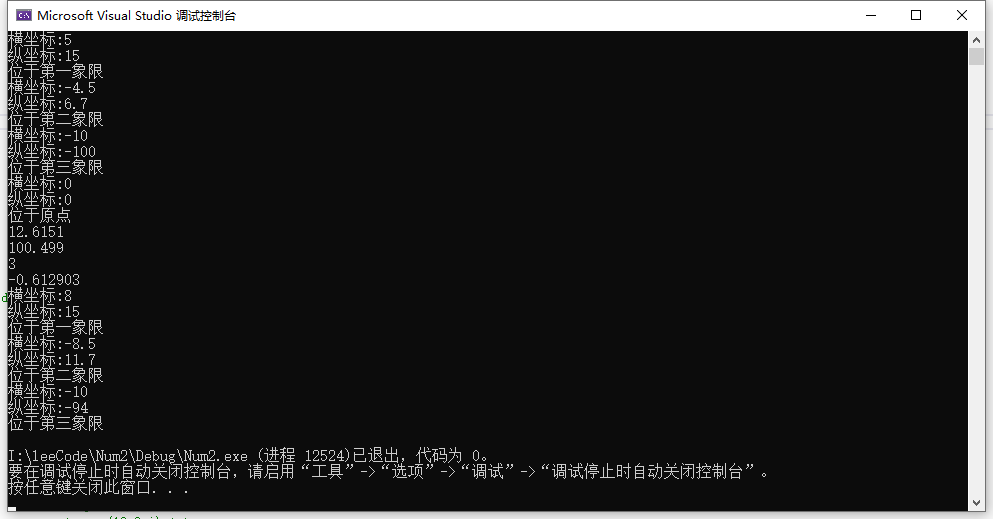2021-06-25 18:39

# 设计并实现一个平面坐标系内的位置类position

❶ 按照描述完成position类的基本的设计和实现。将数据成员设计为私有(private)成员；将成员函数设计为公有(public)成员。并通过以下测试程序。
#include “position.hpp”
#include <iostream>
using namespace std;

int main() {
position a, b, c,d,e;
a.set(5, 15);
a.show();
b.set(-4.5, 6.7);
b.show();
c.set(-10, -100);
c.show();
d.set(20.5, 5.5);
e.set();//默认为原点
e.show();
cout<<distance(a, b)<<endl;
cout<<distance(c)<<endl;//默认求与原点的距离
cout<<a.slope()<<endl;//与原点构成直线的斜率
cout<<a.slope(d)<<endl;    //与d构成直线的斜率
a.move(3);//沿x轴平移
a.show();
b.move(-4, 5);
b.show();
c.move(0, 6);//沿y轴平移
c.show();
return 0;
}

将完整的源代码和测试截图 粘贴在下面。

• 写回答
• 关注问题
• 收藏
• 邀请回答

#### 1条回答默认 最新

•暖晴的天 2021-06-25 19:17
已采纳

其中 position.hpp 文件设计如下：

#include "math.h"
#include <iostream>
using namespace std;
class position
{
public:
position();
~position();

void set(float x = 0 , float y = 0);
float getX() { return this->x; }
float getY() { return this->y; }
void show();
//static float distance(position A, position B = position());
float slope(position A = position());
void move(float x = 0, float y = 0);
private:
float x;
float y;
};

position::position()
{
this->x = 0.0;
this->y = 0.0;
}

position::~position()
{

}

void position::set(float x, float y)
{
this->x = x;
this->y = y;
}

void position::show()
{
cout << "横坐标:" << this->x << endl;
cout << "纵坐标:" << this->y << endl;

if (x == 0 && y == 0)
{
cout << "位于原点" << endl;
}
if (this->x > 0 && this->y > 0)
{
cout << "位于第一象限" << endl;
}
if (this->x < 0 && this->y > 0)
{
cout << "位于第二象限" << endl;
}
if (this->x < 0 && this->y < 0)
{
cout << "位于第三象限" << endl;
}
if (this->x > 0 && this->y < 0)
{
cout << "位于第四象限" << endl;
}
}

float position::slope(position A)
{
return (this->y - A.y) / (this->x - A.x);
}

void position::move(float x, float y)
{
this->x -= x;
this->y -= y;
}

float distance(position A, position B = position());

float distance(position A, position B)
{
return sqrt(pow(2, (A.getX() - B.getX())) + pow(2, (A.getY() - B.getY())));
}

运行结果如下：已采纳该答案
打赏 评论